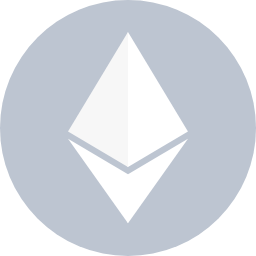#Contract 0x3757b49d79063e157dc376f2b409c3730fa17f61

## Contract Overview

Balance:
0 ETH

ETH Value:
\$0.00

Token:

My Name Tag:
Not Available

Latest 15 from a total of 15 transactions

Txn Hash Method
From
To
Value [Txn Fee]
0x8a6fd1bed20adf8213e12ff5eef1f961d3cc76b1cfdbe8219d1401aa8a586055Release3 days 13 hrs ago0xd8502a71790a2143e336aa2ba915b8851fdfdc3e IN  0x3757b49d79063e157dc376f2b409c3730fa17f610 ETH0.00121553249 ETH
0xa846ce96ee0f90f666534f1fee2fe0e29bb1473bfa4c9057ea954b459b5d0d60Add Beneficiary5 days 5 hrs ago0xde485812e28824e542b9c2270b6b8ed9232b7d0b IN  0x3757b49d79063e157dc376f2b409c3730fa17f610 ETH0.001809336385 ETH
0x6c3cf6ef5319dcb5c6be2bc229a608c8dddd747e2d3bb2a7a7697080a2b96e49Add Beneficiary5 days 5 hrs ago0xde485812e28824e542b9c2270b6b8ed9232b7d0b IN  0x3757b49d79063e157dc376f2b409c3730fa17f610 ETH0.001814053075 ETH
0x0279bb8261ba843c6d146ba24ec2807946e9ecd073253ccedc51b185f9891f37Add Beneficiary5 days 5 hrs ago0xde485812e28824e542b9c2270b6b8ed9232b7d0b IN  0x3757b49d79063e157dc376f2b409c3730fa17f610 ETH0.00181091395 ETH
0x1153c8a17abe994b575efc6f0c6b2ce46ae315f03ef0a037e6b8cc57cb515af0Release6 days 11 hrs ago0xe507c28b1ff52574d8ed1314d96ce7f5e3a95609 IN  0x3757b49d79063e157dc376f2b409c3730fa17f610 ETH0.000688087235 ETH
0x1cce115ea09943932cfd6358d6f11357079330742d1d9be7cd03307434767a70Release7 days 8 hrs ago0x57b3cc13ea26f442d3bcd3fd6cc2bedbca675961 IN  0x3757b49d79063e157dc376f2b409c3730fa17f610 ETH0.001177817344 ETH
0x7507e1c10a7d35552545311c2c44469034e0d8ae3c61625662523ecf2fddbf2fAdd Beneficiary9 days 6 hrs ago0xde485812e28824e542b9c2270b6b8ed9232b7d0b IN  0x3757b49d79063e157dc376f2b409c3730fa17f610 ETH0.002003190173 ETH
0xfa98ed58796bb55149a44a5c74b18eadb2faf9e5de83e09d14c276207d5db806Release10 days 2 hrs ago0xfecca0b07133288fd8a3293d9c3c3eabb3db31c2 IN  0x3757b49d79063e157dc376f2b409c3730fa17f610 ETH0.001424014158 ETH
0x74b520b7edeb31747acd1194bf5c638f3a77dfe1b73ae84dc6a0258c0fbbba4dRelease10 days 4 hrs ago0xb354fe945842b0660111d1700bf2515e40a273d8 IN  0x3757b49d79063e157dc376f2b409c3730fa17f610 ETH0.001409957576 ETH
0x997a0f4ba2beaf4f61c6b0e65ac2d846e9b810b226aa229e06d0476036c36d68Release10 days 4 hrs ago0xe507c28b1ff52574d8ed1314d96ce7f5e3a95609 IN  0x3757b49d79063e157dc376f2b409c3730fa17f610 ETH0.001409966574 ETH
0xcde308bfa3c70eba184be827b8bda800f095101a0d62b02fbd3aa3f393c171feAdd Beneficiary10 days 8 hrs ago0xde485812e28824e542b9c2270b6b8ed9232b7d0b IN  0x3757b49d79063e157dc376f2b409c3730fa17f610 ETH0.002029777425 ETH
0xa1efd1657ecd5fb802b7101a101057e3dafcc0f963e6ea23e5060a7511e62823Add Beneficiary10 days 8 hrs ago0xde485812e28824e542b9c2270b6b8ed9232b7d0b IN  0x3757b49d79063e157dc376f2b409c3730fa17f610 ETH0.002031493117 ETH
0x3f585b013b9e9ef09ab21c1049b4717fb41b495fb37d932309ac065e54424672Add Beneficiary10 days 8 hrs ago0xde485812e28824e542b9c2270b6b8ed9232b7d0b IN  0x3757b49d79063e157dc376f2b409c3730fa17f610 ETH0.002545879042 ETH
0xcc41ebd74aa33f33e84d6ba055aa3b2e33a2d98aec747cd5c1da3171a6d3eee50x6080604012 days 1 hr ago0xde485812e28824e542b9c2270b6b8ed9232b7d0b IN  Contract Creation0 ETH0.084541507685 ETH
Parent Txn Hash Block From To Value### Contract Source Code Verified (Exact Match)

Contract Name:
TeamVestingV2

Compiler Version
v0.7.6+commit.7338295f

Optimization Enabled:
No with 200 runs

Other Settings:
default evmVersion

#### Contract Source Code (Solidity Standard Json-Input format)

File 1 of 7 : TeamVestingV2.sol
File 2 of 7 : IERC20.sol
File 3 of 7 : SafeERC20.sol
File 4 of 7 : SafeMath.sol
File 5 of 7 : Ownable.sol
File 6 of 7 : Address.sol
File 7 of 7 : Context.sol
Settings

#### Contract ABI

`[{"inputs":[{"internalType":"address","name":"_tokenAddress","type":"address"}],"stateMutability":"nonpayable","type":"constructor"},{"anonymous":false,"inputs":[{"indexed":false,"internalType":"address","name":"beneficiary","type":"address"},{"indexed":false,"internalType":"uint256","name":"amount","type":"uint256"},{"indexed":false,"internalType":"uint256","name":"initialReleaseAmount","type":"uint256"},{"indexed":false,"internalType":"uint256","name":"duration","type":"uint256"},{"indexed":false,"internalType":"uint256","name":"startTime","type":"uint256"}],"name":"AddBeneficiary","type":"event"},{"anonymous":false,"inputs":[{"indexed":true,"internalType":"address","name":"previousOwner","type":"address"},{"indexed":true,"internalType":"address","name":"newOwner","type":"address"}],"name":"OwnershipTransferred","type":"event"},{"anonymous":false,"inputs":[{"indexed":false,"internalType":"address","name":"beneficiary","type":"address"}],"name":"RemoveBeneficiary","type":"event"},{"anonymous":false,"inputs":[{"indexed":false,"internalType":"address","name":"beneficiary","type":"address"},{"indexed":false,"internalType":"uint256","name":"amount","type":"uint256"}],"name":"TokensReleased","type":"event"},{"inputs":[{"internalType":"address","name":"_beneficiary","type":"address"},{"internalType":"uint256","name":"_amount","type":"uint256"},{"internalType":"uint256","name":"_initialReleaseAmount","type":"uint256"},{"internalType":"uint256","name":"_duration","type":"uint256"},{"internalType":"uint256","name":"_startTime","type":"uint256"}],"name":"addBeneficiary","outputs":[{"internalType":"bool","name":"","type":"bool"}],"stateMutability":"nonpayable","type":"function"},{"inputs":[],"name":"noOfBeneficiaries","outputs":[{"internalType":"uint256","name":"","type":"uint256"}],"stateMutability":"view","type":"function"},{"inputs":[],"name":"owner","outputs":[{"internalType":"address","name":"","type":"address"}],"stateMutability":"view","type":"function"},{"inputs":[{"internalType":"address","name":"beneficiary","type":"address"}],"name":"releasableAmount","outputs":[{"internalType":"uint256","name":"","type":"uint256"}],"stateMutability":"view","type":"function"},{"inputs":[{"internalType":"address","name":"beneficiary","type":"address"}],"name":"release","outputs":[{"internalType":"uint256","name":"unreleased","type":"uint256"}],"stateMutability":"nonpayable","type":"function"},{"inputs":[{"internalType":"address","name":"_beneficiary","type":"address"}],"name":"removeBeneficiary","outputs":[{"internalType":"bool","name":"","type":"bool"}],"stateMutability":"nonpayable","type":"function"},{"inputs":[],"name":"renounceOwnership","outputs":[],"stateMutability":"nonpayable","type":"function"},{"inputs":[],"name":"token","outputs":[{"internalType":"contract IERC20","name":"","type":"address"}],"stateMutability":"view","type":"function"},{"inputs":[{"internalType":"address","name":"newOwner","type":"address"}],"name":"transferOwnership","outputs":[],"stateMutability":"nonpayable","type":"function"},{"inputs":[{"internalType":"address","name":"beneficiary","type":"address"}],"name":"vestedAmount","outputs":[{"internalType":"uint256","name":"","type":"uint256"}],"stateMutability":"view","type":"function"},{"inputs":[{"internalType":"address","name":"","type":"address"}],"name":"vestedBeneficiaries","outputs":[{"internalType":"uint256","name":"amount","type":"uint256"},{"internalType":"uint256","name":"released","type":"uint256"},{"internalType":"uint256","name":"initialReleaseAmount","type":"uint256"},{"internalType":"uint256","name":"duration","type":"uint256"},{"internalType":"uint256","name":"startTime","type":"uint256"}],"stateMutability":"view","type":"function"}]`

#### Contract Creation Code

`608060405234801561001057600080fd5b50604051620021e4380380620021e48339818101604052602081101561003557600080fd5b810190808051906020019092919050505060006100566101c260201b60201c565b9050806000806101000a81548173ffffffffffffffffffffffffffffffffffffffff021916908373ffffffffffffffffffffffffffffffffffffffff1602179055508073ffffffffffffffffffffffffffffffffffffffff16600073ffffffffffffffffffffffffffffffffffffffff167f8be0079c531659141344cd1fd0a4f28419497f9722a3daafe3b4186f6b6457e060405160405180910390a350600073ffffffffffffffffffffffffffffffffffffffff168173ffffffffffffffffffffffffffffffffffffffff16141561017b576040517f08c379a0000000000000000000000000000000000000000000000000000000008152600401808060200182810382526028815260200180620021bc6028913960400191505060405180910390fd5b80600160006101000a81548173ffffffffffffffffffffffffffffffffffffffff021916908373ffffffffffffffffffffffffffffffffffffffff160217905550506101ca565b600033905090565b611fe280620001da6000396000f3fe608060405234801561001057600080fd5b50600436106100a95760003560e01c806359633a1c1161007157806359633a1c14610256578063715018a6146102b05780638da5cb5b146102ba578063bfb8aea9146102ee578063f2fde38b14610362578063fc0c546a146103a6576100a9565b80631726cbc8146100ae57806319165587146101065780632edef73b1461015e578063384711cc1461017c57806355119b1a146101d4575b600080fd5b6100f0600480360360208110156100c457600080fd5b81019080803573ffffffffffffffffffffffffffffffffffffffff1690602001909291905050506103da565b6040518082815260200191505060405180910390f35b6101486004803603602081101561011c57600080fd5b81019080803573ffffffffffffffffffffffffffffffffffffffff169060200190929190505050610440565b6040518082815260200191505060405180910390f35b6101666105e8565b6040518082815260200191505060405180910390f35b6101be6004803603602081101561019257600080fd5b81019080803573ffffffffffffffffffffffffffffffffffffffff1690602001909291905050506105ee565b6040518082815260200191505060405180910390f35b61023e600480360360a08110156101ea57600080fd5b81019080803573ffffffffffffffffffffffffffffffffffffffff169060200190929190803590602001909291908035906020019092919080359060200190929190803590602001909291905050506108a2565b60405180821515815260200191505060405180910390f35b6102986004803603602081101561026c57600080fd5b81019080803573ffffffffffffffffffffffffffffffffffffffff169060200190929190505050610dd8565b60405180821515815260200191505060405180910390f35b6102b8611284565b005b6102c26113f1565b604051808273ffffffffffffffffffffffffffffffffffffffff16815260200191505060405180910390f35b6103306004803603602081101561030457600080fd5b81019080803573ffffffffffffffffffffffffffffffffffffffff16906020019092919050505061141a565b604051808681526020018581526020018481526020018381526020018281526020019550505050505060405180910390f35b6103a46004803603602081101561037857600080fd5b81019080803573ffffffffffffffffffffffffffffffffffffffff169060200190929190505050611450565b005b6103ae611642565b604051808273ffffffffffffffffffffffffffffffffffffffff16815260200191505060405180910390f35b6000610439600260008473ffffffffffffffffffffffffffffffffffffffff1673ffffffffffffffffffffffffffffffffffffffff1681526020019081526020016000206001015461042b846105ee565b61166890919063ffffffff16565b9050919050565b600061044b826103da565b9050600081116104a6576040517f08c379a0000000000000000000000000000000000000000000000000000000008152600401808060200182810382526023815260200180611f476023913960400191505060405180910390fd5b6104fb81600260008573ffffffffffffffffffffffffffffffffffffffff1673ffffffffffffffffffffffffffffffffffffffff168152602001908152602001600020600101546116eb90919063ffffffff16565b600260008473ffffffffffffffffffffffffffffffffffffffff1673ffffffffffffffffffffffffffffffffffffffff1681526020019081526020016000206001018190555061058e8282600160009054906101000a900473ffffffffffffffffffffffffffffffffffffffff1673ffffffffffffffffffffffffffffffffffffffff166117739092919063ffffffff16565b7fc7798891864187665ac6dd119286e44ec13f014527aeeb2b8eb3fd413df931798282604051808373ffffffffffffffffffffffffffffffffffffffff1681526020018281526020019250505060405180910390a1919050565b60035481565b600080600260008473ffffffffffffffffffffffffffffffffffffffff1673ffffffffffffffffffffffffffffffffffffffff168152602001908152602001600020600001549050600260008473ffffffffffffffffffffffffffffffffffffffff1673ffffffffffffffffffffffffffffffffffffffff1681526020019081526020016000206004015442101561068a57600091505061089d565b610721600260008573ffffffffffffffffffffffffffffffffffffffff1673ffffffffffffffffffffffffffffffffffffffff16815260200190815260200160002060030154600260008673ffffffffffffffffffffffffffffffffffffffff1673ffffffffffffffffffffffffffffffffffffffff168152602001908152602001600020600401546116eb90919063ffffffff16565b4210610730578091505061089d565b6000610787600260008673ffffffffffffffffffffffffffffffffffffffff1673ffffffffffffffffffffffffffffffffffffffff168152602001908152602001600020600201548361166890919063ffffffff16565b9050610898600260008673ffffffffffffffffffffffffffffffffffffffff1673ffffffffffffffffffffffffffffffffffffffff1681526020019081526020016000206002015461088a600260008873ffffffffffffffffffffffffffffffffffffffff1673ffffffffffffffffffffffffffffffffffffffff1681526020019081526020016000206003015461087c61086d600260008b73ffffffffffffffffffffffffffffffffffffffff1673ffffffffffffffffffffffffffffffffffffffff168152602001908152602001600020600401544261166890919063ffffffff16565b8661181590919063ffffffff16565b61189b90919063ffffffff16565b6116eb90919063ffffffff16565b925050505b919050565b60006108ac611924565b73ffffffffffffffffffffffffffffffffffffffff166108ca6113f1565b73ffffffffffffffffffffffffffffffffffffffff1614610953576040517f08c379a00000000000000000000000000000000000000000000000000000000081526004018080602001828103825260208152602001807f4f776e61626c653a2063616c6c6572206973206e6f7420746865206f776e657281525060200191505060405180910390fd5b600073ffffffffffffffffffffffffffffffffffffffff168673ffffffffffffffffffffffffffffffffffffffff1614156109d9576040517f08c379a0000000000000000000000000000000000000000000000000000000008152600401808060200182810382526030815260200180611e776030913960400191505060405180910390fd5b60008511610a32576040517f08c379a000000000000000000000000000000000000000000000000000000000815260040180806020018281038252602d815260200180611e4a602d913960400191505060405180910390fd5b838511610a8a576040517f08c379a0000000000000000000000000000000000000000000000000000000008152600401808060200182810382526042815260200180611edb6042913960600191505060405180910390fd5b6000600260008873ffffffffffffffffffffffffffffffffffffffff1673ffffffffffffffffffffffffffffffffffffffff1681526020019081526020016000206000015414610b25576040517f08c379a0000000000000000000000000000000000000000000000000000000008152600401808060200182810382526034815260200180611ea76034913960400191505060405180910390fd5b60008311610b7e576040517f08c379a000000000000000000000000000000000000000000000000000000000815260040180806020018281038252602a815260200180611e20602a913960400191505060405180910390fd5b42821015610bd7576040517f08c379a0000000000000000000000000000000000000000000000000000000008152600401808060200182810382526037815260200180611da26037913960400191505060405180910390fd5b81600260008873ffffffffffffffffffffffffffffffffffffffff1673ffffffffffffffffffffffffffffffffffffffff1681526020019081526020016000206004018190555082600260008873ffffffffffffffffffffffffffffffffffffffff1673ffffffffffffffffffffffffffffffffffffffff1681526020019081526020016000206003018190555084600260008873ffffffffffffffffffffffffffffffffffffffff1673ffffffffffffffffffffffffffffffffffffffff1681526020019081526020016000206000018190555083600260008873ffffffffffffffffffffffffffffffffffffffff1673ffffffffffffffffffffffffffffffffffffffff16815260200190815260200160002060020181905550610d42333087600160009054906101000a900473ffffffffffffffffffffffffffffffffffffffff1673ffffffffffffffffffffffffffffffffffffffff1661192c909392919063ffffffff16565b610d5860016003546116eb90919063ffffffff16565b6003819055507f517ecd3643be5d9960179aabf8f498ceaf117c84138d0612164195a3cb4a31948686868686604051808673ffffffffffffffffffffffffffffffffffffffff1681526020018581526020018481526020018381526020018281526020019550505050505060405180910390a16001905095945050505050565b6000610de2611924565b73ffffffffffffffffffffffffffffffffffffffff16610e006113f1565b73ffffffffffffffffffffffffffffffffffffffff1614610e89576040517f08c379a00000000000000000000000000000000000000000000000000000000081526004018080602001828103825260208152602001807f4f776e61626c653a2063616c6c6572206973206e6f7420746865206f776e657281525060200191505060405180910390fd5b600073ffffffffffffffffffffffffffffffffffffffff168273ffffffffffffffffffffffffffffffffffffffff161415610f0f576040517f08c379a0000000000000000000000000000000000000000000000000000000008152600401808060200182810382526030815260200180611e776030913960400191505060405180910390fd5b6000600260008473ffffffffffffffffffffffffffffffffffffffff1673ffffffffffffffffffffffffffffffffffffffff168152602001908152602001600020600001541415610fab576040517f08c379a0000000000000000000000000000000000000000000000000000000008152600401808060200182810382526043815260200180611f6a6043913960600191505060405180910390fd5b6000610fb6836103da565b1115610fc757610fc582610440565b505b6110aa33611062600260008673ffffffffffffffffffffffffffffffffffffffff1673ffffffffffffffffffffffffffffffffffffffff16815260200190815260200160002060010154600260008773ffffffffffffffffffffffffffffffffffffffff1673ffffffffffffffffffffffffffffffffffffffff1681526020019081526020016000206000015461166890919063ffffffff16565b600160009054906101000a900473ffffffffffffffffffffffffffffffffffffffff1673ffffffffffffffffffffffffffffffffffffffff166117739092919063ffffffff16565b6000600260008473ffffffffffffffffffffffffffffffffffffffff1673ffffffffffffffffffffffffffffffffffffffff168152602001908152602001600020600401819055506000600260008473ffffffffffffffffffffffffffffffffffffffff1673ffffffffffffffffffffffffffffffffffffffff168152602001908152602001600020600301819055506000600260008473ffffffffffffffffffffffffffffffffffffffff1673ffffffffffffffffffffffffffffffffffffffff168152602001908152602001600020600001819055506000600260008473ffffffffffffffffffffffffffffffffffffffff1673ffffffffffffffffffffffffffffffffffffffff168152602001908152602001600020600201819055506000600260008473ffffffffffffffffffffffffffffffffffffffff1673ffffffffffffffffffffffffffffffffffffffff16815260200190815260200160002060010181905550611228600160035461166890919063ffffffff16565b6003819055507f49ed5a425b7b67ea44959f73b24f6619d66c387d763a7217b9dc7980801b44b082604051808273ffffffffffffffffffffffffffffffffffffffff16815260200191505060405180910390a160019050919050565b61128c611924565b73ffffffffffffffffffffffffffffffffffffffff166112aa6113f1565b73ffffffffffffffffffffffffffffffffffffffff1614611333576040517f08c379a00000000000000000000000000000000000000000000000000000000081526004018080602001828103825260208152602001807f4f776e61626c653a2063616c6c6572206973206e6f7420746865206f776e657281525060200191505060405180910390fd5b600073ffffffffffffffffffffffffffffffffffffffff1660008054906101000a900473ffffffffffffffffffffffffffffffffffffffff1673ffffffffffffffffffffffffffffffffffffffff167f8be0079c531659141344cd1fd0a4f28419497f9722a3daafe3b4186f6b6457e060405160405180910390a360008060006101000a81548173ffffffffffffffffffffffffffffffffffffffff021916908373ffffffffffffffffffffffffffffffffffffffff160217905550565b60008060009054906101000a900473ffffffffffffffffffffffffffffffffffffffff16905090565b60026020528060005260406000206000915090508060000154908060010154908060020154908060030154908060040154905085565b611458611924565b73ffffffffffffffffffffffffffffffffffffffff166114766113f1565b73ffffffffffffffffffffffffffffffffffffffff16146114ff576040517f08c379a00000000000000000000000000000000000000000000000000000000081526004018080602001828103825260208152602001807f4f776e61626c653a2063616c6c6572206973206e6f7420746865206f776e657281525060200191505060405180910390fd5b600073ffffffffffffffffffffffffffffffffffffffff168173ffffffffffffffffffffffffffffffffffffffff161415611585576040517f08c379a0000000000000000000000000000000000000000000000000000000008152600401808060200182810382526026815260200180611d7c6026913960400191505060405180910390fd5b8073ffffffffffffffffffffffffffffffffffffffff1660008054906101000a900473ffffffffffffffffffffffffffffffffffffffff1673ffffffffffffffffffffffffffffffffffffffff167f8be0079c531659141344cd1fd0a4f28419497f9722a3daafe3b4186f6b6457e060405160405180910390a3806000806101000a81548173ffffffffffffffffffffffffffffffffffffffff021916908373ffffffffffffffffffffffffffffffffffffffff16021790555050565b600160009054906101000a900473ffffffffffffffffffffffffffffffffffffffff1681565b6000828211156116e0576040517f08c379a000000000000000000000000000000000000000000000000000000000815260040180806020018281038252601e8152602001807f536166654d6174683a207375627472616374696f6e206f766572666c6f77000081525060200191505060405180910390fd5b818303905092915050565b600080828401905083811015611769576040517f08c379a000000000000000000000000000000000000000000000000000000000815260040180806020018281038252601b8152602001807f536166654d6174683a206164646974696f6e206f766572666c6f77000000000081525060200191505060405180910390fd5b8091505092915050565b6118108363a9059cbb60e01b8484604051602401808373ffffffffffffffffffffffffffffffffffffffff16815260200182815260200192505050604051602081830303815290604052907bffffffffffffffffffffffffffffffffffffffffffffffffffffffff19166020820180517bffffffffffffffffffffffffffffffffffffffffffffffffffffffff83818316178352505050506119ed565b505050565b6000808314156118285760009050611895565b600082840290508284828161183957fe5b0414611890576040517f08c379a0000000000000000000000000000000000000000000000000000000008152600401808060200182810382526021815260200180611dff6021913960400191505060405180910390fd5b809150505b92915050565b6000808211611912576040517f08c379a000000000000000000000000000000000000000000000000000000000815260040180806020018281038252601a8152602001807f536166654d6174683a206469766973696f6e206279207a65726f00000000000081525060200191505060405180910390fd5b81838161191b57fe5b04905092915050565b600033905090565b6119e7846323b872dd60e01b858585604051602401808473ffffffffffffffffffffffffffffffffffffffff1681526020018373ffffffffffffffffffffffffffffffffffffffff1681526020018281526020019350505050604051602081830303815290604052907bffffffffffffffffffffffffffffffffffffffffffffffffffffffff19166020820180517bffffffffffffffffffffffffffffffffffffffffffffffffffffffff83818316178352505050506119ed565b50505050565b6000611a4f826040518060400160405280602081526020017f5361666545524332303a206c6f772d6c6576656c2063616c6c206661696c65648152508573ffffffffffffffffffffffffffffffffffffffff16611adc9092919063ffffffff16565b9050600081511115611ad757808060200190516020811015611a7057600080fd5b8101908080519060200190929190505050611ad6576040517f08c379a000000000000000000000000000000000000000000000000000000000815260040180806020018281038252602a815260200180611f1d602a913960400191505060405180910390fd5b5b505050565b6060611aeb8484600085611af4565b90509392505050565b606082471015611b4f576040517f08c379a0000000000000000000000000000000000000000000000000000000008152600401808060200182810382526026815260200180611dd96026913960400191505060405180910390fd5b611b5885611c9c565b611bca576040517f08c379a000000000000000000000000000000000000000000000000000000000815260040180806020018281038252601d8152602001807f416464726573733a2063616c6c20746f206e6f6e2d636f6e747261637400000081525060200191505060405180910390fd5b6000808673ffffffffffffffffffffffffffffffffffffffff1685876040518082805190602001908083835b60208310611c195780518252602082019150602081019050602083039250611bf6565b6001836020036101000a03801982511681845116808217855250505050505090500191505060006040518083038185875af1925050503d8060008114611c7b576040519150601f19603f3d011682016040523d82523d6000602084013e611c80565b606091505b5091509150611c90828286611caf565b92505050949350505050565b600080823b905060008111915050919050565b60608315611cbf57829050611d74565b600083511115611cd25782518084602001fd5b816040517f08c379a00000000000000000000000000000000000000000000000000000000081526004018080602001828103825283818151815260200191508051906020019080838360005b83811015611d39578082015181840152602081019050611d1e565b50505050905090810190601f168015611d665780820380516001836020036101000a031916815260200191505b509250505060405180910390fd5b939250505056fe4f776e61626c653a206e6577206f776e657220697320746865207a65726f20616464726573735465616d56657374696e6756323a2053746172742074696d652063616e6e6f74206265206265666f72652063757272656e742074696d65416464726573733a20696e73756666696369656e742062616c616e636520666f722063616c6c536166654d6174683a206d756c7469706c69636174696f6e206f766572666c6f775465616d56657374696e6756323a204475726174696f6e207061737365642063616e6e6f7420626520305465616d56657374696e6756323a20416d6f756e742073686f756c64206265206c6172676572207468616e20305465616d56657374696e6756323a2042656e65666963696172792063616e6e6f742062652061203020616464726573735465616d56657374696e6756323a2043616e6e6f7420616464207468652073616d652062656e656669636961727920616761696e5465616d56657374696e6756323a20416d6f756e742073686f756c64206265206c6172676572207468616e20696e697469616c2072656c6561736520616d6f756e745361666545524332303a204552433230206f7065726174696f6e20646964206e6f7420737563636565645465616d56657374696e6756323a204e6f2072656c65617361626c6520616d6f756e745465616d56657374696e6756323a2043616e6e6f742072656d6f766520612062656e656669636961727920776869636820686173206e6f74206265656e206164646564a264697066735822122064a53c61d4fd565ef338ffaade96688b06f5265834b37fb705deeedb0a5c6c4c64736f6c634300070600335465616d56657374696e6756323a20546f6b656e20616464726573732063616e6e6f7420626520300000000000000000000000006c2c06790b3e3e3c38e12ee22f8183b37a13ee55`

#### Constructor Arguments (ABI-Encoded and is the last bytes of the Contract Creation Code above)

`0000000000000000000000006c2c06790b3e3e3c38e12ee22f8183b37a13ee55-----Decoded View---------------Arg  : _tokenAddress (address): 0x6c2c06790b3e3e3c38e12ee22f8183b37a13ee55-----Encoded View---------------1 Constructor Arguments found :Arg  : 0000000000000000000000006c2c06790b3e3e3c38e12ee22f8183b37a13ee55`

Block Transaction Gas Used Reward